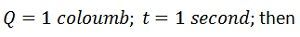What is meant by Electrical Current

What is meant by Electrical Current?

Definition: Electric current is defined as the rate of flow of negative charges of the conductor. In other words, the continuous flow of electrons in an electric circuit is called an electric current.The conducting material consists a large number of free electrons which move from one atom to the other at random.

Electrical current is the flow rate of electric charge in electric field, usually in electrical circuit.

Using water pipe analogy, we can visualize the electrical current as water current that flows in a pipe.

The electrical current is measured in ampere (amp) unit.

Unit of Current

Since the charge is measured in coulombs and time in seconds, so the unit of electric current is coulomb/Sec (C/s) or amperes (A). The amperes is the SIunit of the conductor. The I is the symbolic representation of the current.Thus, a wire is said to carry a current of one ampere when charge flows through it at the rate of one coulomb per second.

When an electrical potential difference is applied across the metallic wire, the loosely attached free electrons start moving towards the positive terminal of the cell shown in the figure below. This continuous flow of electrons constitutes the electrical current. The flow of currents in the wire is from the negative terminal of the cell to the positive terminal through the external circuit.

Electrical Voltage

Kirchoff Law

Resistance

Ohm’s Law

Ampere

Difference between Voltage and Current